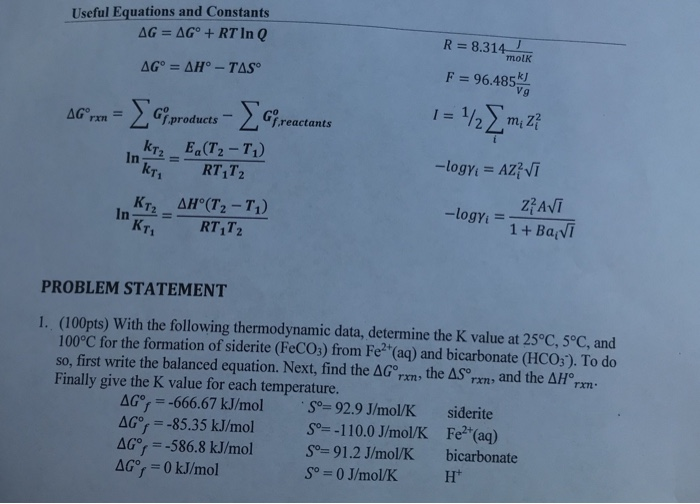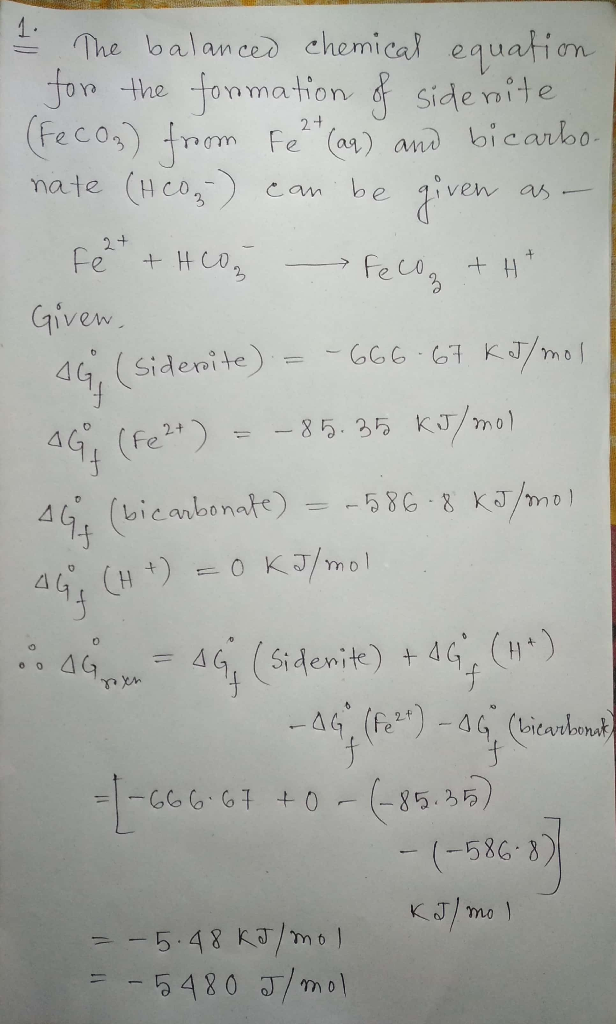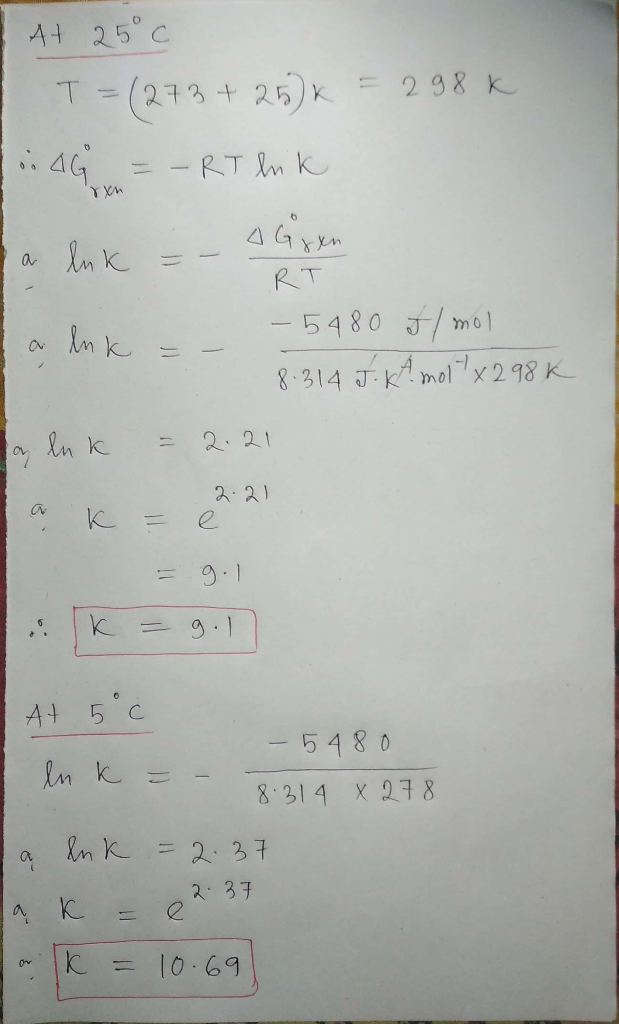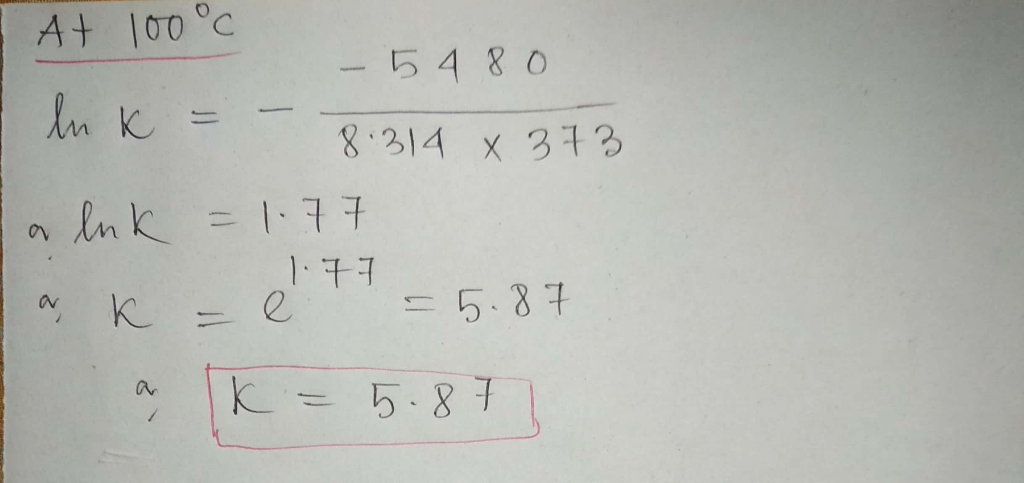Homework Help Question & Answers

# Useful Equations and Constants AG AG RTIn Q R 8.314olk F96485% vg > .G7,reactants 22, m Zi Ink互=...Useful Equations and Constants AG AG RTIn Q R 8.314olk F96485% vg > .G7,reactants 22, m Zi Ink互= Ea(T2-T1) 112 ΔΗ'(T2-T1) In- T1 =-T1T2 PROBLEM STATEMENT (100pts) With the following thermodynamic data, determine the K value at 25°C, 5°C, and 100°C for the formation of siderite (FeCOs) from Fe2 (aq) and bicarbonate (HCO;). To do so, first write the balanced equation. Next, find the ΔGorm, the ASorm, and the ΔΗ0rm. Finally give the K value for each temperature. 1. ΔG。--666.67 kJ/mol . So-929 J/mol/K siderite AG 85.35 kJ/mol110.0 J/mol/K dGor =-586.8 kJ/mol Soー91.2 J/mol/K Fe (aq) bicarbonate Ht So 0 J/mol/K

#### Homework Answers

ReportAnswer #1Add Homework Help Answer
##### Add Answer of: Useful Equations and Constants AG AG RTIn Q R 8.314olk F96485% vg > .G7,reactants 22, m Zi Ink互=...
Your Answer: Your Name: What's your source?
Not the answer you're looking for? Ask your own homework help question. Our experts will answer your question WITHIN MINUTES for Free.
More Homework Help Questions Additional questions in this topic.

• #### Explain the equation of respiration and describe the reactants and products in terms of where they come from, how they are produced, and what they do in the reaction

Need Online Homework Help?

Get FREE EXPERT Answers
WITHIN MINUTES
Related Questions